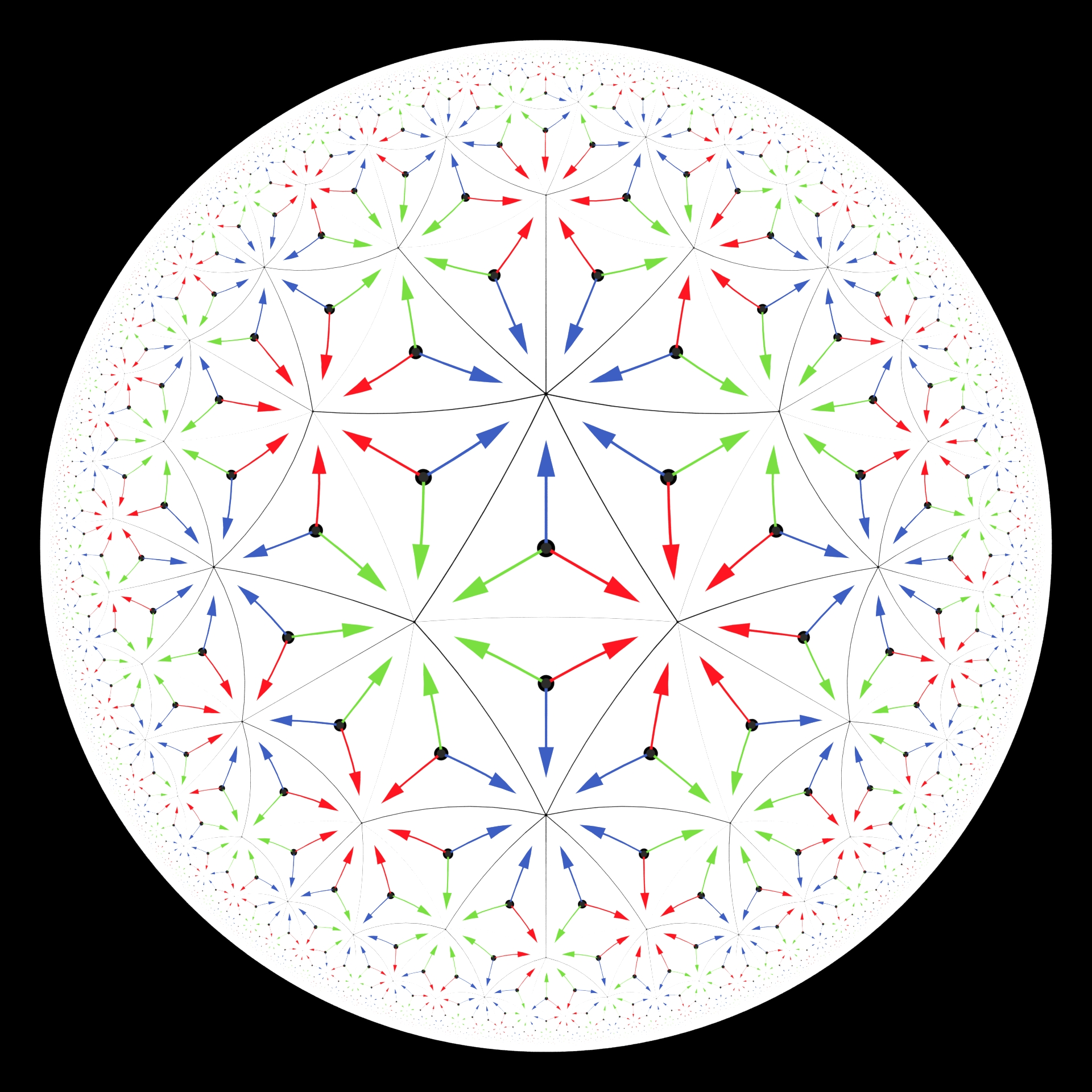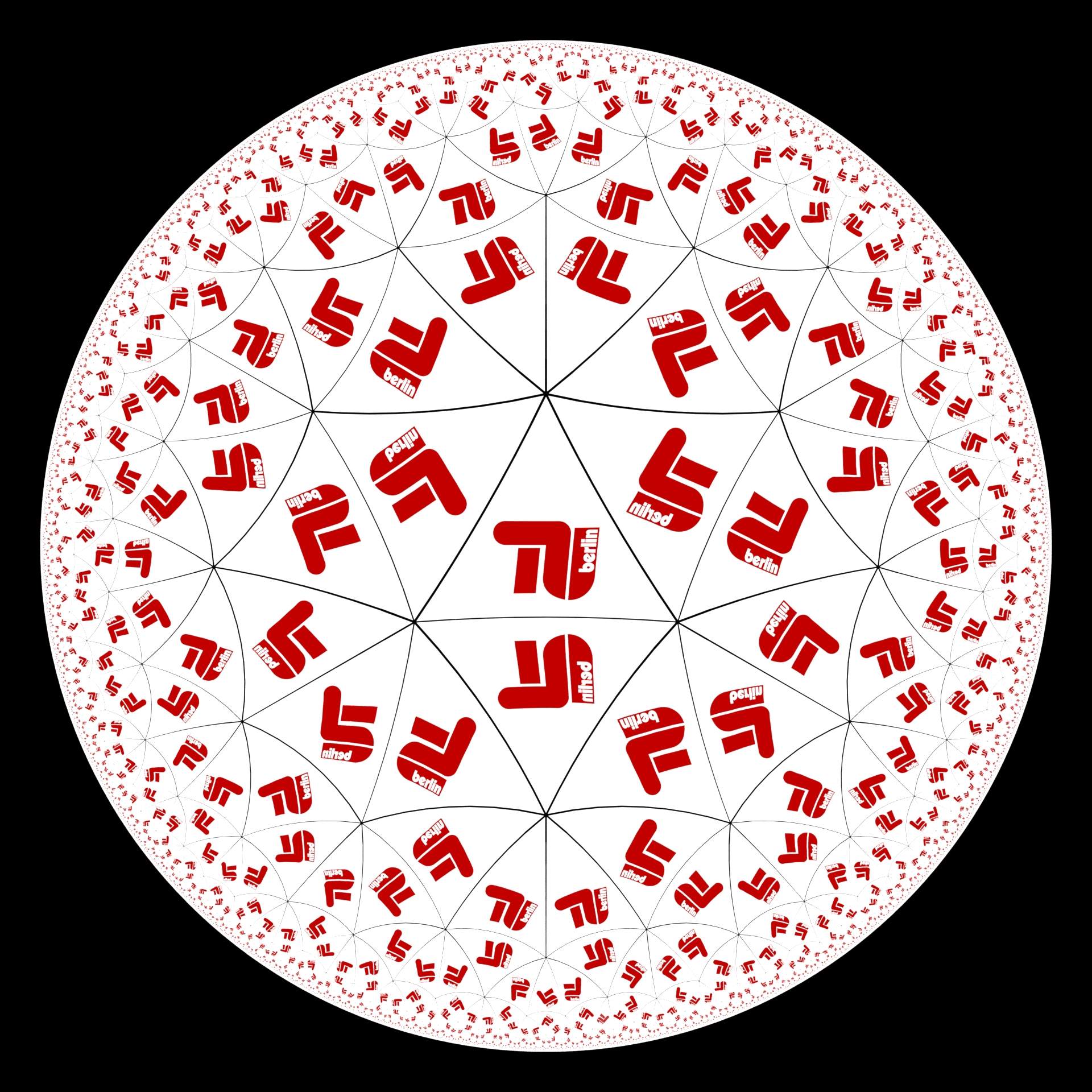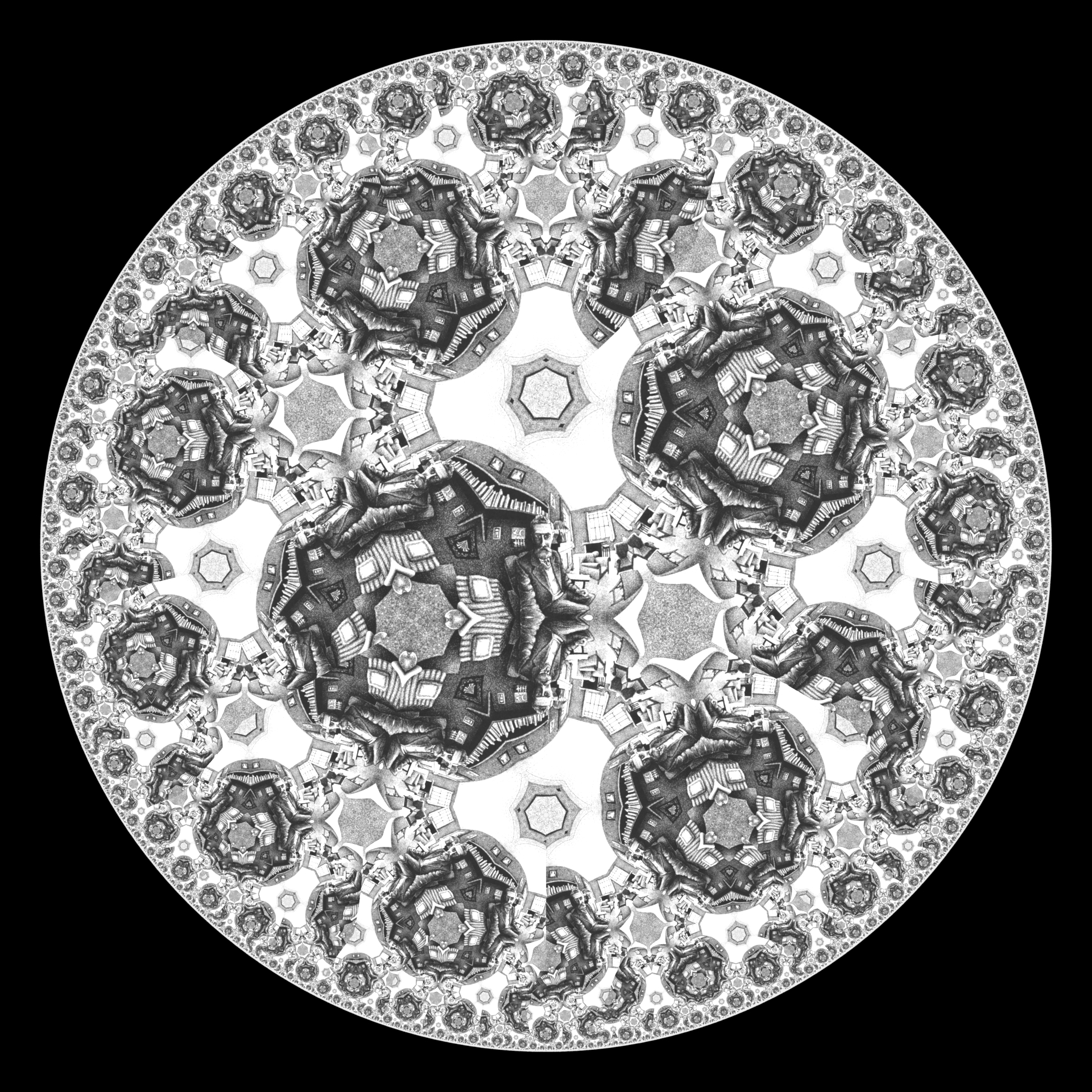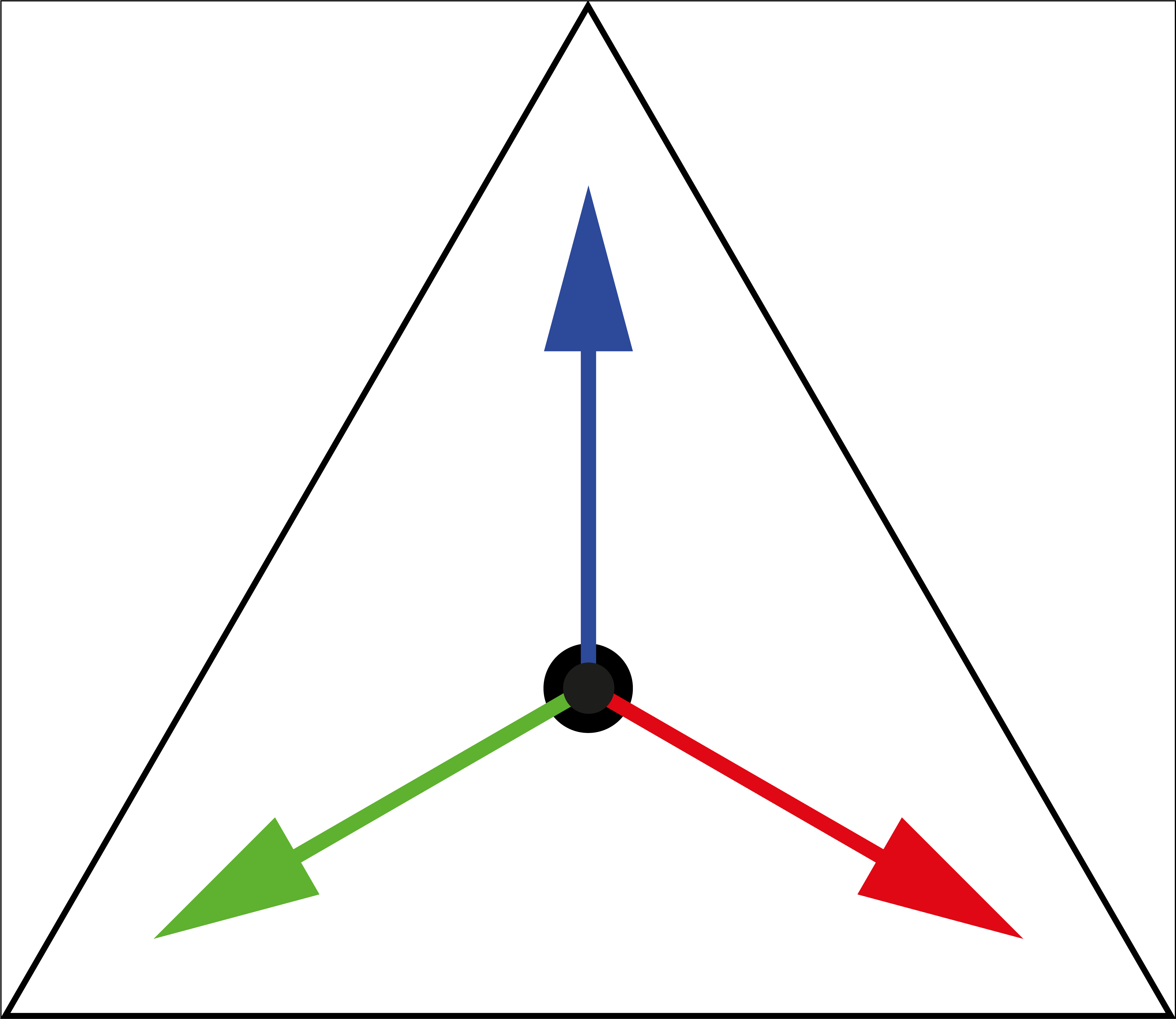📐Hyperbolic Tiling {3,7}

# Hyperbolic Tiling {3,7}

A private side project

A slice of the hyperbolic tiling.Tiling of the poincare disc model using arrow triangles, a tringle with the TU Berlin logo, and an image of M.C. Escher.

## Intro

Here are some documentations on my attempt to generate a hyperbolic tiling. The goal was to set up a system where I can use any texture/video to be displayed inside the tiling. With a bit of creativity, we could then make images in the style of M.C. Escher’s circle limit

## Mission Statement:

Given any texture of a triangle in the Klein disc model, ……map it into each triangle of the {3,7} tiling of the Poincaré disk model.## Solution

• Create the basic reference mesh for the tiling
• Create a single base triangle in the center with only 3 points.
• For each edge:
• Check if the edge-normal is pointing away.
• If so, reflect the opposite point along the edge using circle inversion.
• Mark edges to avoid reflecting twice.
• This should Create a tiling with some overlaps.
• Delete the overlappers at each iteration.
• Create the refined textured mesh of a single tile
• Take the single base triangle mentioned above
• Transform Poincaré-disc model -> Klein-disc model.
• Remesh the triangle.
• Orthogonally map uv coordinates onto it.
• Transform Klein-disc model -> Poincaré-disc model.
• Expand the refined tile using the reference mesh. Iteratively for each edge of the reference mesh
• Reflect the points on one side of the edge to the other side using circle inversion of a circle that runs through both points and hits the unit circle orthogonally.
• After each reflection, mark the edge to avoid reflecting twice.
• For the fine details at the edge
• Place a circle of constant color equal to the average color of a tile behind the tiling.
• Be aware of the following:
• When using floating points the numerics of the tiny triangles near the edge become unstable. Testing for linear independence of two vectors that are extremely close to each other can be difficult.

## Results

Notice that the arrow’s colors don’t match up everywhere. There is not much freedom when trying to build a seamless pattern.

This image can serve as a template to fill with different images. This triangle will then be mapped to the entire tiling.

The triangle arrow covering shows that in order to get a seamless pattern, we need every triangle edge to fit each other triangle edge, even when flipped. This is a necessity for the {3,7} tiling. When we try using a normal image we are likely doomed to have bad edge alignments somewhere. There will be triangles with non-unique and non-fitting arrangements depending on the reflection-path taken to get there during the creation. Here we use a self-portrait from M.C. Escher where you can see where two triangles meet at some non-continuous boarders.An image of Escher in the tiling. The overlap-edge reflection discontinuity is hard to see in this mess.

Using the TU logo we get this:TU Berlin logo tiling.

If we stick to radially symmetric textures we can get quite fancy and get smooth images. Here is some fitting music . Put this on fullscreen and stare at it for a minute.

4k 60fps version of a hyperbolic tiling with a periodic color triangle at each tile.

The clip that was placed into each triangle.

## Notes

There is already a web version of a good tiling generator, but it does not allow us to place the initial image in the straight Klein model setting. These sources were very inspiring for this work.
🔗 Make Hyperbolic Tilings of Images
🔗 Interactive Hyperbolic Tiling in the Poincaré Disc